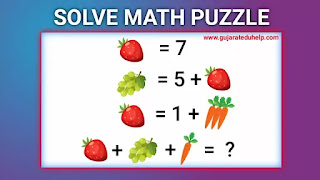## Wednesday, November 23, 2022

### Math Puzzle with Answer - Puzzle for Kids No - 1

Math Puzzle with Answer - Puzzle for Kids No - 1

Math Puzzle with Answer - Puzzle for Kids No - 1
Welcome to Gujarat Eduhelp puzzles

Can you solve this high IQ math puzzle?Hello and welcome to my  blog  We have planned for students and teachers to participate in the Gujarat Eduhelp puzzle. Knowledge growth is our motto. Nowadays online education is necessary for everyone, but we have so many problems getting an online education. Here we have to do our best to give general knowledge to students and teachers.

Number Puzzle with the answer - Puzzle for children
Today we are facing many problems to get homeschooling, but online education is the best opportunity to update our knowledge. we have to try to impart general knowledge by planning puzzles for children.

A puzzle is a game, problem, or toy that tests a person's ingenuity or knowledge. In a puzzle, the solver has to put the pieces together in a logical way to arrive at the correct or fun solution of the puzzle. There are different kinds of puzzles such as crossword puzzles, word search puzzles, number puzzles, relationship puzzles, and logic puzzles.

For More Puzzles:

Puzzles are often created as a form of entertainment, but they can also result from serious math or logic problems. In such cases, your solution can make a significant contribution to mathematical research.

Puzzle solutions often require recognizing patterns and following a particular type of order. People with a high level of inductive reasoning skills may be more adept at solving these puzzles than others. But puzzles based on inquiry and discovery can be more easily solved by those with good deduction skills.

Deductive reasoning improves with practice. Math puzzles often involve BODMAS. BODMAS is an acronym and stands for Bracket, Of, Division, Multiplication, Addition, and Subtraction. In some areas, PEDMAS (parentheses, exponents, division, multiplication, addition, and subtraction) is synonymous with BODMAS. Explain the order of operations to solve an expression.

Some math puzzles require top-down convention to avoid ambiguity in the order of operations. It's an elegantly simple idea that relies, like Sudoku does, on the requirement that numbers appear only once, starting from top to bottom.

🍓 = 7

🍇 = 5 +🍓

🍓 = 1 +🥕🥕🥕

🍓+ 🍇 +🥕 = ?

Explanation:

Suppose, 🍓=x, 🍇=y, 🥕=z

x = 7

y = 5 + x
y = 5 + 7
so, y = 12

x = 1 + 3z
7 = 1 + 3z
3z = 7 - 1
3z = 6
so, z = 2

x + y + z = ?
7 + 12 + 2 = 21